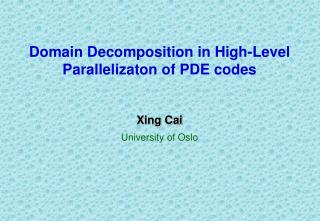DownloadDownload PresentationDomain Decomposition in High-Level Parallelizaton of PDE codes

# Domain Decomposition in High-Level Parallelizaton of PDE codes

Télécharger la présentation## Domain Decomposition in High-Level Parallelizaton of PDE codes

- - - - - - - - - - - - - - - - - - - - - - - - - - - E N D - - - - - - - - - - - - - - - - - - - - - - - - - - -
##### Presentation Transcript

1. Domain Decomposition in High-Level Parallelizaton of PDE codes Xing Cai University of Oslo

2. Outline of the Talk • Introduction and motivation • A simulator parallel model • A generic programming framework • Applications

3. The Question Starting point: sequential PDE simulators. How to do the parallelization? • Resulting parallel simulators should have • Good parallel performance • Good overall numerical performance • A relative simple parallelization process Inroduction • We need • a good parallelization strategy • a good implementation of the strategy

4. 3 Key Words • Parallel Computing • faster solution, larger simulation • Domain Decomposition (additive Schwarz method) • good algorithmic efficiency • mathematical foundation of parallelization • Object-Oriented Programming • extensible sequential simulator • flexible implementation framework for parallelization Introduction

5. A Known Problem • “The hope among early domain decomposition workers was that one could write a simple controlling program which would call the old PDE software directly to perform the subdomain solves. This turned out to be unrealistic because most PDE packages are too rigid and inflexible.” • - Smith, Bjørstad and Gropp • The remedy: • Correct use of object-oriented programming techniques. Introduction

6. Additive Schwarz Method Example: Solving the Poisson problem on the unit square Domain Decomposition

7. Parallelization • A simulator-parallel model • Each processor hosts an arbitrary number of subdomains • balance between algorithmic efficiency and load balancing • One subdomain is assigned with a sequential simulator • Flexibility - different linear system solvers, preconditioners, convergence monitors etc. can easily be chosen for different subproblems • Domain decomposition at the level of subdomain simulators! Design

8. The Simulator-Parallel Model • Reuse of existing sequential simulators • Data distribution is implied • No need for global data • Needs additional functionalities for exchanging nodal values inside the overlapping region • Needs some global administration Observations

9. A Generic Programming Framework • An add-on library (SPMD model) • Use of object-oriented programming technique • Flexibility and portability • Simplified parallelization process for end-user OO Implementation

10. The Administrator • Parameter Interface solution method or preconditioner, max iterations, stopping criterion etc • DD algorithm Interface access to predefined numerical algorithme.g.CG • Operation Interface (standard codes & UDC) access to subdomain simulators, matrix-vector product, inner product etc OO Implementation

11. The Communicator • Encapsulation of communication related codes Hidden concrete communication model MPI in use, but easy to change • Communication pattern determination • Inter-processor communication • Intra-processor communication OO Implementation

12. The Subdomain Simulator • Subdomain Simulator -- a generic representation • C++ class hierarchy • Standard interface of generic member functions OO Implementation

13. SubdomainSimulator SubdomainFEMSolver OldSimulator NewSimulator Adaptation of Subdomain Simulator • class NewSimulator : public SubdomainFEMSolver • public OldSimulator • { • // …. • virtual void createLocalMatrix () • { OldSimualtor::makeSystem (); } • }; OO Implementation

14. Performance • Algorithmic efficiency • efficiency of original sequential simulator(s) • efficiency of domain decomposition method • Parallel efficiency • communication overhead (low) • coarse grid correction overhead (normally low) • load balancing • subproblem size • work on subdomain solves

15. Application • Test case: 2D Poisson problem on unit square. • Fixed subdomains M=32 based on a 481 x 481 global grid. • Straightforward parallelization of an existing simulator. • Subdomain solves use CG+FFT Simulator Parallel P: number of processors.

16. Application • Test case: 2D linear elasticity, 241 x 241 global grid. • Vector equation • Straightforward parallelization based on an existing Diffpack simulator Simulator Parallel

17. 2D Linear Elasticity Simulator Parallel

18. 2D Linear Elasticity • P: number of processors in use (P=M). • I: number of parallel BiCGStab iterations needed. • Multigrid V-cycle in subdomain solves Simulator Parallel

19. Unstructured Grid Application

20. Application • Test case: two-phase porous media flow problem. SEQ: PEQ: Simulator Parallel I: average number of parallel BiCGStab iterations per step Multigrid V-cycle in subdomain solves

21. Two-Phase Porous Media Flow Simulator Parallel Simulation result obtained on 16 processors

22. Two-Phase Porous Media Flow

23. Application • Test case: fully nonlinear 3D water wave problem. Simulator Parallel • Parallelization based on an existing Diffpack simulator.

24. Preliminary Results • Fixed number of subdomains M=16. • Subdomain grids from partitioning a global 41x41x41 grid. • Simulation over 32 time steps. • DD as preconditioner of CG for the Laplace eq. • Multigrid V-cycle as subdomain solver. Simulator Parallel

25. 3D Water Waves Simulator Parallel

26. Summary • High-level parallelization of PDE codes through DD • Introduction of a simulator-parallel model • A generic implementation framework Simulator Parallel